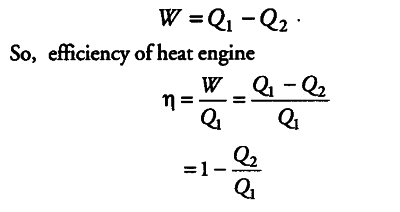# Explain what do you understand by the efficiency of a heat engine?

The efficiency of a heat engine is stated as the ratio of the net work done by the heat engine and heat absorbed by the working substance.
Suppose a heat engine absorbs { Q }_{ 1 } heat from the hot reservoir and gives
{ Q }_{ 2 } heat to the colder reservoir. So, the work done by the working substance is